Sie sind hier: ICP » R. Hilfer » Publikationen

# 2 Mathematical Model

[334.3.1] Let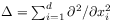denote the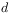-dimensional Laplace operator in cartesian coordinates. [334.3.2] Numerous authors postulate a fractional diffusion equation such as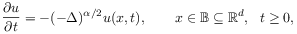(1)

with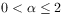and initial condition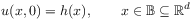(2)

[page 335, §0]    for a function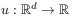as a mathematical model for various physical phenomena (see [10, 15, 14, 28, 29] for examples). [335.0.1] For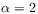this becomes the Cauchy problem for the ordinary diffusion equation whose applicability as a mathematical model for physical phenomena has been validated with innumerable experiments. [335.0.2] For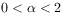however experimental evidence remains narrowly bounded in space and time scales. [335.0.3] Moreover, theoretical considerations cast fundamental doubts on the applicability of this case to natural phenomena.

[335.1.1] For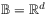the fractional Laplace operator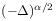in eq. (1) may be defined (in the sense of Riesz ) as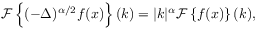(3)

where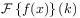denotes the Fourier transform of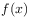. [335.1.2] A core domain suitable for various extensions are functions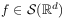from the Schwartz space of smooth functions decreasing rapidly at infinity.

[335.2.1] The implicit idealizing assumption underlying the choice of an unbounded domainin eq. (1) is that the boundary is sufficiently far away so that its effects on the observations are negligible. [335.2.2] However, experiments are normally performed inside a bounded laboratory containing a bounded apparatus that occupies a bounded domain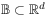of space. [335.2.3] Thus, practical applications require to consider nonlocal boundary value problems on bounded domains.

[335.3.1] Every experiment assumes that the experimental conditions in the region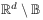surrounding the region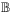containing the sample can be controlled and reproduced to any desired degree of accuracy. [335.3.2] In the mathematical model this is represented by assuming given boundary data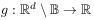for the unknown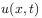such that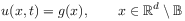(4)

for all times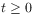. [335.3.3] The Riesz operatormay then be be understood as a Dirichlet form on the space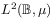over the bounded setequipped with the canonical Borel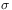-algebra and a-finite measure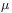, .